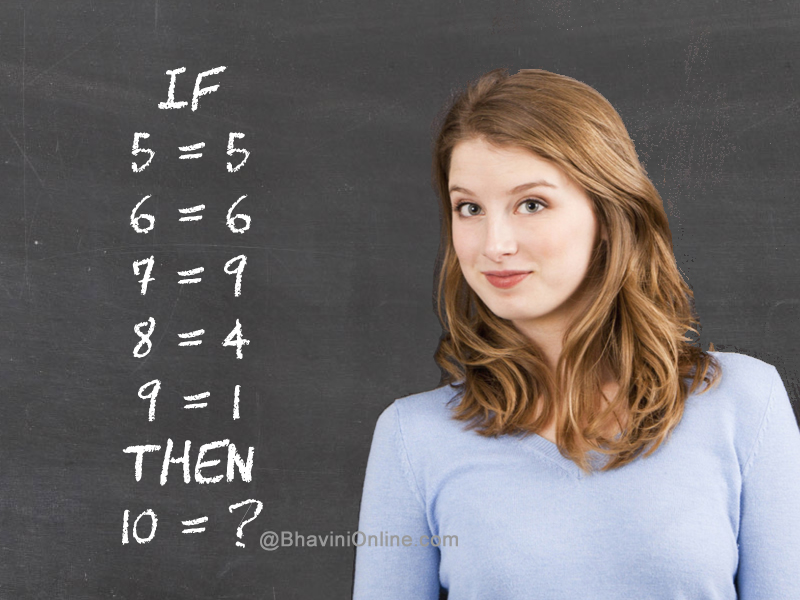# Fun With Numbers Riddle: If 5 = 5 & 6 = 6 Then 10 = ?

Look at the series of equations and find the logic behind the numbers.

Once you find the connection you will be able to find the missing number in the last equation.

If

5 = 5

6 = 6

7 = 9

8 = 4

9 = 1

Then

10 = ?So were you able to solve the riddle? Leave your answers in the comment section below.